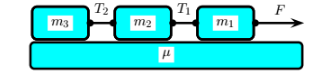Problem: A force F pulls three blocks with masses m 1, m2, and m3 along a surface as shown the the picture below. The coefficient of kinetic friction between the blocks and the surface is given by μ, the tension in the strings between the blocks are T1 and T2, and the acceleration of the blocks is a. The acceleration of gravity is g. What is a correct equation for the tension T2 between the blocks with masses m2 and m3? 1. T2 = m2 (μg − a) − T1 2. T2 = T1 + m2 (μg + a) 3. T2 = T1 − m2 (μg − a) 4. T2 = T1 + m2 (μg) 5. T2 = F + T1 − m2 (μg + a) 6. T2 = T1 − m2 (μg + a) 7. T2 = T1 + m2 (μa) 8. T2 = T1 − m2 (μg) 9. T2 = T1 − m2 (a − μg) 10. T2 = T1 − μm2g + F − m 2a

82% (14 ratings)
Problem Details

A force F pulls three blocks with masses m 1, m2, and m3 along a surface as shown the the picture below. The coefficient of kinetic friction between the blocks and the surface is given by μ, the tension in the strings between the blocks are T1 and T2, and the acceleration of the blocks is a. The acceleration of gravity is g. What is a correct equation for the tension T2 between the blocks with masses m2 and m3?

1. T2 = m2 (μg − a) − T1

2. T2 = T1 + m2 (μg + a)

3. T2 = T1 − m2 (μg − a)

4. T2 = T1 + m2 (μg)

5. T2 = F + T1 − m2 (μg + a)

6. T2 = T1 − m2 (μg + a)

7. T2 = T1 + m2 (μa)

8. T2 = T1 − m2 (μg)

9. T2 = T1 − m2 (a − μg)

10. T2 = T1 − μm2g + F − m 2